## Practical Differentiator:

The noise and stability at high frequency can be corrected, in the practical differentiator circuit using the resistance R1 in series with C1 and the capacitor Cf in parallel with resistance Rf.

The circuit is shown in the Fig. 2.49. The resistance Rcomp is used for bias compensation.### Analysis of the Practical Differentiator:

As the input current of op-amp is zero, there is no current input at node B. Hence it is at the ground potential. From the concept of the virtual ground, node A is also at the ground potential and hence VB = VA = 0V.

For the current I, we can write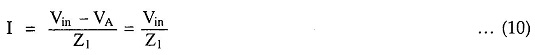where Z1 = R1 in series with C1

So in Laplace domain we can write,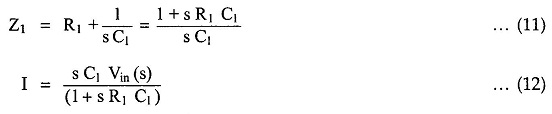Now the current I1 is,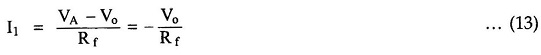In Laplace,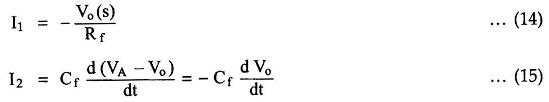Taking we get,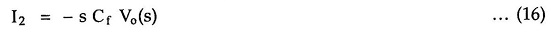Applying at node A,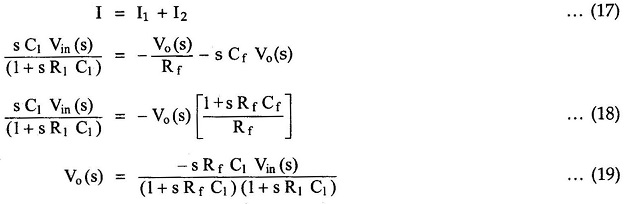If RfCf = R1C1 then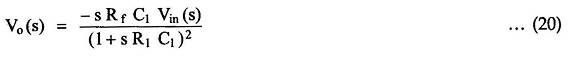The time constant RfC1 is much greater than R1C1 or RfCf and hence the equation (20) reduces to,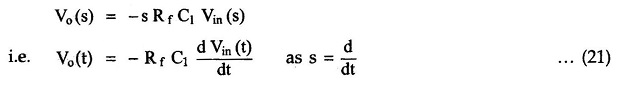Thus the output voltage is the RfC1 times the differentiation of the input.

It may be noted that though RfC1 is much larger than RfCf or R1C1, it is less than or equal to the time period T of the input, for the true differentiation.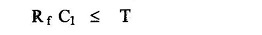### Applications of Practical Differentiator:

The practical differentiator circuits are most commonly used in :

1. In the wave shaping circuits to detect the high frequency components in the input signal.
2. As a rite-of-change detector in the FM demodulators.
3. The differentiator circuit is avoided in the analog computers.
Scroll to Top